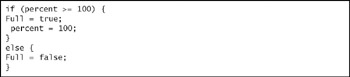As shown in Figure 26.11, both the if and else statements use braces {} to mark the beginning and end of the blocks of code they condition. The else statement is optional. For a complete list of logical operators, see Table 26.3:Figure 26.11: Java application using logical operators.

Notice that the equal test is performed with two equal signs (==). This is because a single equal sign is an assignment, meaning that it assigns a value to the variable to its left. One equal sign cannot be used as a test.

 == Equal != Not Equal < Less than > Greater than <= Less or equal >= Greater or equalIBM i5/iSeries Primer(c) Concepts and Techniques for Programmers, Administrators, and Sys[... ]ators
ISBN: N/A
EAN: N/A
Year: 2004
Pages: 245